# QUESTION 3 How much heat (in kJ) is required to raise the temperature of 122 g of ethanol (mw 46.07) from 11.29 °C to i...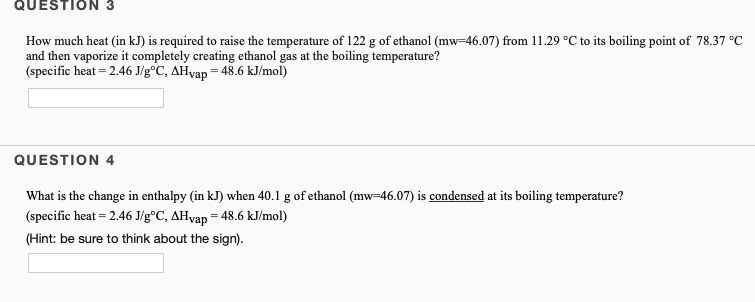QUESTION 3 How much heat (in kJ) is required to raise the temperature of 122 g of ethanol (mw 46.07) from 11.29 °C to its boiling point of 78.37 °C and then vaporize it completely creating ethanol gas at the boiling temperature? (specific heat - 2.46 J/g°C, AHyap = 48.6 kJ/mol) QUESTION 4 What is the change in enthalpy (in kJ) when 40.1 g of ethanol (mw-46.07) is condensed at its boiling temperature? (specific heat 2.46 J/g°C, AHvap 48.6 kJ/mol) (Hint: be sure to think about the sign)

3)

Ti = 11.29 oC

Tf = 78.37 oC

here

Cl = 2.46 J/g.oC

Heat required to convert liquid from 11.29 oC to 78.37 oC

Q1 = m*Cl*(Tf-Ti)

= 122 g * 2.46 J/g.oC *(78.37-11.29) oC

= 20132.0496 J

Hvap = 48.6KJ/mol =

48600J/mol

Lets convert mass to mol

Molar mass of C2H5OH = 46.07 g/mol

number of mol

n= mass/molar mass

= 122.0/46.07

= 2.6483 mol

Heat required to convert liquid to gas at 78.37 oC

Q2 = n*Hvap

= 2.6483 mol *48600 J/mol

= 128705.392 J

Total heat required = Q1 + Q2

= 20132.0496 J + 128705.392 J

= 148837 J

= 148.8 KJ

4)

Molar mass of C2H5OH = 46.07 g/mol

mass(C2H5OH)= 40.1 g

use:

number of mol of C2H5OH,

n = mass of C2H5OH/molar mass of C2H5OH

=(40.1 g)/(46.07 g/mol)

= 0.8705 mol

Given:

ΔH = -48.6 KJ/mol [negative because condensation is opposite of vapourisation]

use:

Q = ΔH * number of mol

= -48.6 KJ/mol * 0.8705 mol

= -42.3 KJ

#### Earn Coin

Coins can be redeemed for fabulous gifts.

Similar Homework Help Questions
• ### How much heat (in kJ) is required to raise the temperature of 202.8 g of ethanol...

How much heat (in kJ) is required to raise the temperature of 202.8 g of ethanol (mw=46.07) from 40.34 °C to its boiling point of  78.37 °C and then vaporize it completely creating ethanol gas at the boiling temperature? (specific heat = 2.46 J/g°C, ΔHvap = 48.6 kJ/mol)

• ### How much heat (in kJ) is required to raise the temperature of 288.7 g of ethanol...

How much heat (in kJ) is required to raise the temperature of 288.7 g of ethanol (mw=46.07) from 39.38 °C to its boiling point of 78.37 °C and then vaporize it completely creating ethanol gas at the boiling temperature? (specific heat = 2.46 J/g°C, ΔHvap = 48.6 kJ/mol)

• ### How much heat (in kJ) is required to raise the temperature of 124.9 g of ethanol...

How much heat (in kJ) is required to raise the temperature of 124.9 g of ethanol (mw=46.07) from 30.24 °C to its boiling point of  78.37 °C and then vaporize it completely creating ethanol gas at the boiling temperature? (specific heat = 2.46 J/g°C, ΔHvap = 48.6 kJ/mol)

• ### QUESTION 3 How much heat (in kJ) is required to raise the temperature of 141,4 g...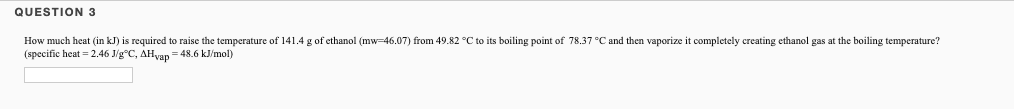QUESTION 3 How much heat (in kJ) is required to raise the temperature of 141,4 g of ethanol (mw-46,07) from 49.82 °C to its boiling point of 78.37 °C and then vaporize it completely creating ethanol gas at the boiling temperature? (specific heat=2.46 J/gºC, AH yap = 48.6 kJ/mol)

• ### a. How much heat (kJ), at 1 atm, is required to raise the temperature of 25.0...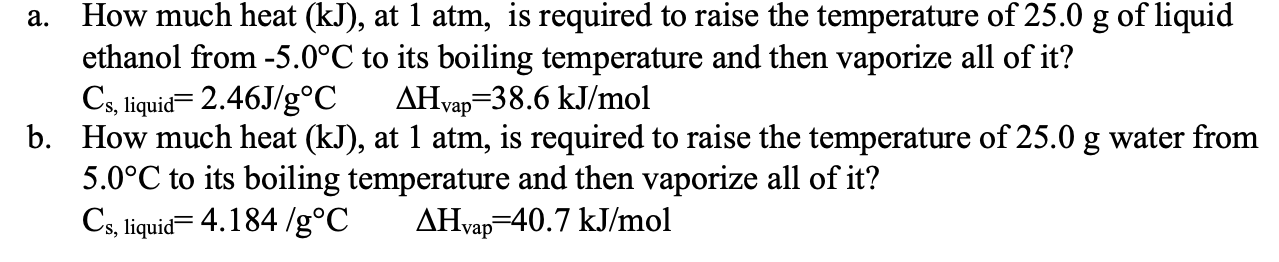a. How much heat (kJ), at 1 atm, is required to raise the temperature of 25.0 g of liquid ethanol from -5.0°C to its boiling temperature and then vaporize all of it? Cs, liquid= 2.46J/g°C AHvap=38.6 kJ/mol b. How much heat (kJ), at 1 atm, is required to raise the temperature of 25.0 g water from 5.0°C to its boiling temperature and then vaporize all of it? Cs, liquid=4.184 /g°C AHvap=40.7 kJ/mol

• ### Calculate the amount of heat (in kJ) required to raise the temperature of 14.0 g of...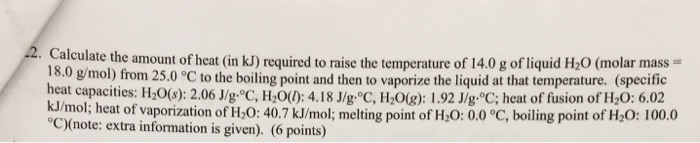Calculate the amount of heat (in kJ) required to raise the temperature of 14.0 g of liquid H_2O (molar mass = 18.0 g/mol) from 25.0 degree C to the boiling point and then to vaporize the liquid at that temperature, (specific heal capacities: H_2O(s): 2.06 J/g degree C, H_2O(l): 4.18 J/g degree C, H_2O(g): 1.92 J/g degree C; heat of fusion of H_2O: 6.02 kJ/mol; heat of vaporization of H_2O: 40.7 kJ/mol; melting point of H_2O: 0.0 degree C, boiling...

• ### Determine the amount of heat energy (in kJ) needed to warm a 58.5-g sample of ethanol...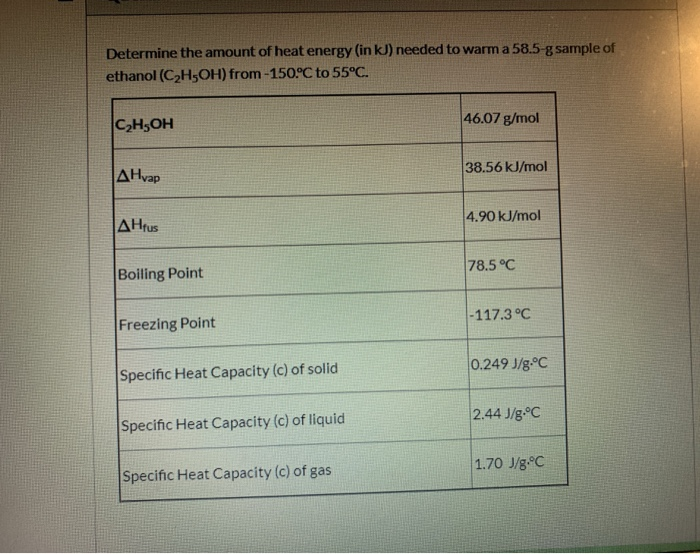Determine the amount of heat energy (in kJ) needed to warm a 58.5-g sample of ethanol (C2H5OH) from-150°C to 55°C. C2H5OH 46.07 g/mol AHvap 38.56 kJ/mol ΔΗrus 4.90 kJ/mol Boiling Point 78.5°C Freezing Point -117.3°C Specific Heat Capacity (c) of solid 0.249 J/g.°C Specific Heat Capacity (c) of liquid 2.44 J/g.°C Specific Heat Capacity (c) of gas 1.70 J/8.°C

• ### Given the following information for ethanol, C,H,OH (at 1 atm), calculate the amount of heat in kneeded (at 1 atm)...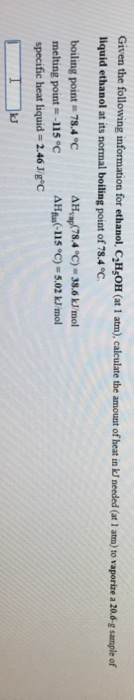Given the following information for ethanol, C,H,OH (at 1 atm), calculate the amount of heat in kneeded (at 1 atm) to vaporize a 20.6-g sample of liquid ethanol at its normal boiling point of 78.4 °C. boiling point = 78.4 °C AHvap 78.4 °C) = 38.6 kJ/mol melting point = -115°C AH(-115 °C) -5.02 kJ/mol specific heat liquid = 2.46 J/gºC

• ### Select all accurate statements about the heating curve shown for ethanol (C)H,OH). C2H5OH 46.07 g/mol 161...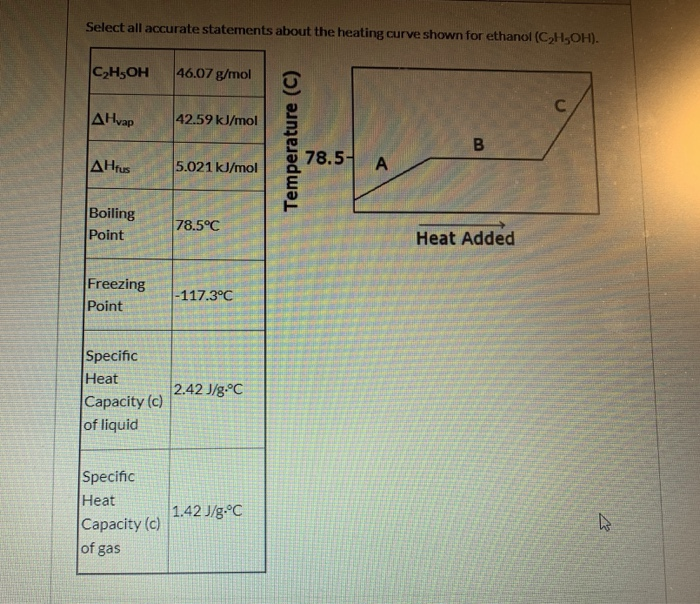Select all accurate statements about the heating curve shown for ethanol (C)H,OH). C2H5OH 46.07 g/mol 161 C AHvap 42.59KJ/mol 78.5 AHfus A 5.021 kJ/mol Boiling Point 78.5°C Heat Added Freezing Point -117.3°C Specific Heat Capacity (c) of liquid 2.42 J/g°C Specific Heat 1.42 J/g°C Capacity (c) of gas Temperature (C) B The portion of the heating curve labeled "A represents the increase in the average kinetic energy of the liquid phase of ethanol. The portion of the heating curve labeled...

• ### How much energy is required to raise the temperature of 33.6 g of water from -25.5°C to 86.4°C

How much energy is required to raise the temperature of 33.6 g of water from -25.5°C to 86.4°C?melting point 0.0°C boiling point 100.0°C specific heat solid 2.05 J/g · °C specfic heat liquid 4.184 J/g · °C specific heat gas 2.02 J/g · °C H° fusion 6.02 kJ/mol H° vaporization 40.7 kJ/mol

Free Homework App